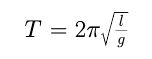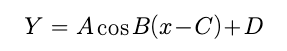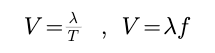# Short note on Wave Motion - Simple Harmonic Motion

Simple Harmonic Motion

A repeating motion in which the acceleration is directly related to the displacement (distance away from the equilibrium) and always directed towards equilibrium.Cosine CurvesWhere

A = amplitude ( 0) : how much energy it has

CosB = period ( 2 PIE / t) : time, 1 oscillation

C = horz. Shift : human error

D = vert. Shift : distance, to x-axis

Waves

Graphed SHM, transfer of energy

Vibration : WORK to get energy

Propagates : what energy moves through

Mechanical (light)

Needs a medium

More dense – better Mechanical

Electromagnetic (sound)

does NOT need a medium

less dense – better

Mechanical Waves

Transverse : medium vibrates perp. to energy
Most common ex. Guitar string, slinky

Longitudinal : medium vibrates para. to energy Has compressions ex:

sound Surface : both para. and perp. to energy “physics bob” ex: earthquakes, waves

Principle of SuperpositionStanding Waves

A continuous wave train of equal amplitude (RAD), wavelength (m), and frequ. (Hz) (/sec) in the same medium creating nodes and antinodes.

Boundary : change in medium (part of energy gets reflected, part gets absorbed)

rigidity : how much energy gets ABSORBED

close rigidity -> more absorbed
different rigidity -> more reflected

Interference in Diffraction

Crest + crest = antinode

Crest + troph = node

Post By : Rahul Kumar 28 Mar, 2020 1599 views Physics